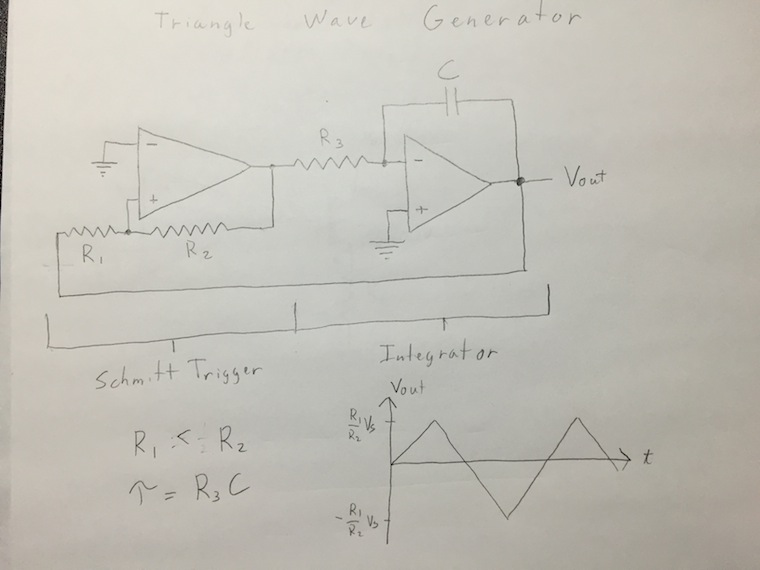Date Series Part 2 of fading hat

A long time ago I wrote a post about a hat born out of the frustration of all the blinking I saw at burning man. This hat would not blink, but slowly fade the LEDs on and off in a less seizure inducing pattern. I think though, "I will tell the world about this hat!" I started ambitiously with a video introducing circuits. I spent time editing this video together. I posted the first video on youtube, and then I got tired and didn't get back to it.

Now, I have decided to do away with constraining notions like "video quality" and "editing" and have completed the video. Now you can make this circuit.

Below is the four part series of videos starting with basic circuits and moving to the final triangle wave generator which powers the fading LED hat. I've also included a final bonus video of another person's hat which uses the same circuit and is much better than my simple hat. It's 72 minutes of wild breadboarding action.

Below is the key diagram showing the triangle wave generator circuit. In my case I set the resistance $$R_{1}$$ to 47 k$$\Omega$$, $$R_{2}$$ to 100 k$$\Omega$$, $$R_{3}$$ to 10 M$$\Omega$$, and $$C$$ to 0.1 $$\mu$$F.